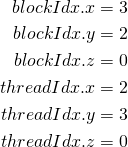# CUDA – Dimensions, Mapping and Indexing

## Kernel Invocation

The host calls a kernel using a triple chevron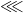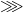. In the chevrons we place the number of blocks and the number of threads per block.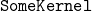100, 256would launch 100 blocks of 256 threads each (total of 25600 threads).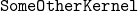50, 1024would launch 50 blocks of 1024 threads each (51200 threads in total).

## Dimensions

As many parallel applications involve multidimensional data, it is convenient to organize thread blocks into 1D, 2D or 3D arrays of threads. The blocks in a grid must be able to be executed independently, as communication or cooperation between blocks in a grid is not possible. When a kernel is launched the number of threads per thread block, and the number of thread blocks is specified, this, in turn, defines the total number of CUDA threads launched. For example if the maximum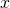,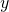and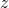dimensions of a block are 512, 512 and 64, it should be allocated such that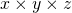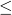512, which is the maximum number of threads per block. The limitation on the number of threads in a block is actually imposed because the number of registers that can be allocated across all threads is limited. Blocks can be organized into one- or two-dimensional grids (say up to 65,535 blocks) in each dimension.

dim3 is a 3d structure or vector type with three integers,,and. One can initialise as many of the three coordinates as they like

   dim3 threads(256);           // Initialise with x as 256, y and z will both be 1
dim3 blocks(100, 100);       // Initialise x and y, z will be 1
dim3 anotherOne(10, 54, 32); // Initialises all three values, x will be 10, y gets 54 and z will be 32.

## Mapping

Every thread in CUDA is associated with a particular index so that it can calculate and access memory locations in an array.

Each of the above are dim3 structures and can be read in the kernel to assign particular workloads to any thread.

## Indexing

Here is an example indexing scheme based on the mapping defined above.

The grid (on the left) has size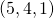, that is, it has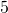blocks in thedirection,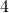blocks in thedirection, andblock in thedirection.

Each block (on the right) is of size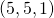withthreads along theanddirections, andthread along thedirection.

At the grid level (on the left), the tuple for each block is the 3D index, e.g.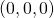, and below the 3D index is the 1D index of each block, e.g.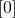.

At the block level (on the right), a similar indexing scheme applies, where the tuple is the 3D index of the thread within the block and the number in the square bracket is the 1D index of the thread within the block.

During execution, the CUDA threads are mapped to the problem in an undefined manner. Randomly completed threads and blocks are shown as green to highlight the fact that the order of execution for threads is undefined.

There are a total of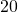blocks in a grid. Each block has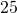threads for a total of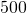threads. Here are the steps to find the indices for a particular thread, say thread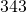. This number has to be expressed in terms of the block size.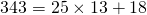With respect to 0-indexing, the 17th thread of the 13th block is thread.

From the figure, the 13th block maps to the coordinates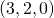and the 17th thread maps to the coordinates. Thus threadis indexed by